# 4-DETERMINANTS

Here we are going through the ncert solutions for class 12 maths chapter 4- determinants so before going through the ncert solutions make sure to go through the textbook that helps you to understand the solutions more easily

#### Question 1:

Evaluate the determinants in Exercises 1 and 2.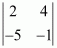= 2(−1) − 4(−5) = − 2 + 20 = 18

#### Question 2:

Evaluate the determinants in Exercises 1 and 2.

(i)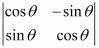(ii)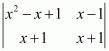(i)= (cos θ)(cos θ) − (−sin θ)(sin θ) = cos2 θ+ sin2 θ = 1

(ii)= (x2 − x + 1)(x + 1) − (x − 1)(x + 1)

x3 − x2 + x + x2 − x + 1 − (x2 − 1)

x3 + 1 − x2 + 1

x3 − x2 + 2

#### Question 3:

If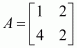, then show that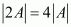The given matrix is.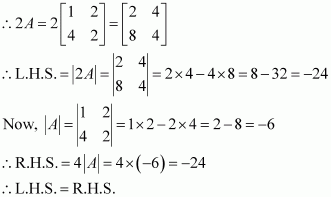#### Question 4:

If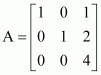, then show that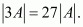The given matrix is.

It can be observed that in the first column, two entries are zero. Thus, we expand along the first column (C1) for easier calculation.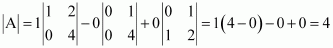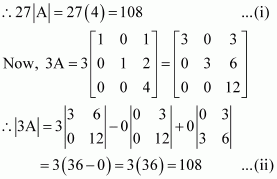From equations (i) and (ii), we have: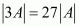Hence, the given result is proved.

#### Question 5:

Evaluate the determinants

(i)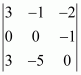(iii)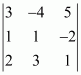(ii)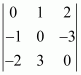(iv)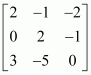(i) Let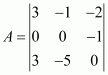.

It can be observed that in the second row, two entries are zero. Thus, we expand along the second row for easier calculation.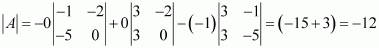(ii) Let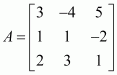.

By expanding along the first row, we have: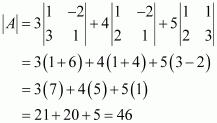(iii) Let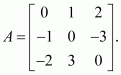By expanding along the first row, we have: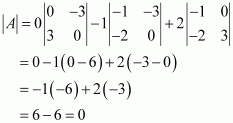(iv) Let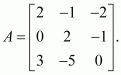By expanding along the first column, we have: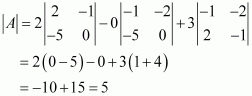#### Question 6:

If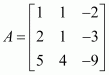, find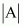.

Let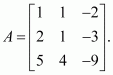By expanding along the first row, we have: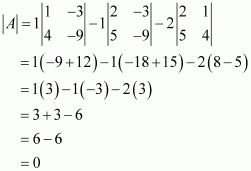#### Question 7:

Find values of x, if

(i) ∣∣∣2541∣∣∣=∣∣∣2x64x∣∣∣2451=2x46x

(ii)
∣∣∣2435∣∣∣=∣∣∣x2x35∣∣∣2345=x32x5

(i)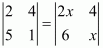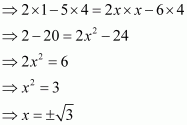(ii)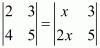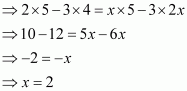#### Question 8:

If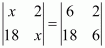, then x is equal to

(A) 6 (B) ±6 (C) −6 (D) 0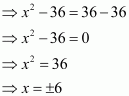Hence, the correct answer is B.

​​ ​​ ​​ ​​ ​​ ​​ ​​ ​​ ​​ ​​ ​​ ​​ ​​ ​​ ​​ ​​ ​​ ​​ ​​ ​​ ​​ ​​ ​​ ​​ ​​ ​​ ​​ ​​ ​​ ​​ ​​ ​​ ​​ ​​ ​​ ​​ ​​ ​​ ​​ ​​ ​​ ​​ ​​ ​​ ​​ ​​ ​​ ​​ ​​ ​​ ​​ ​​ ​​ ​​ ​​ ​​ ​​ ​​ ​​ ​​ ​​ ​​ ​​ ​​ ​​ ​​ ​​ ​​ ​​ ​​ ​​ ​​ ​​ ​​ ​​ ​​ ​​ ​​ ​​ ​​ ​​ ​​ ​​ ​​​​ EXERCISE 4.2

Question 1:

Using the property of determinants and without expanding, prove that: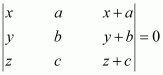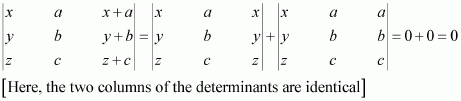#### Question 2:

Using the property of determinants and without expanding, prove that: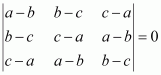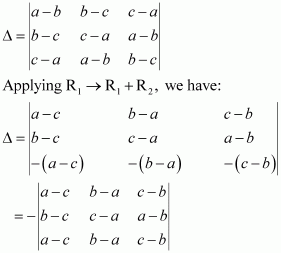Here, the two rows R1 and R3 are identical.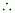Δ = 0.

#### Question 3:

Using the property of determinants and without expanding, prove that: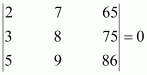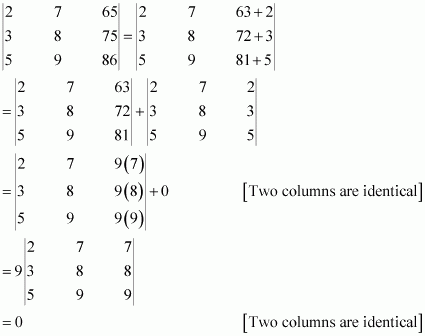#### Question 4:

Using the property of determinants and without expanding, prove that: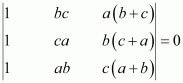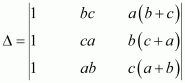By applying C→ C3 + C2, we have: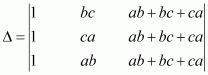Here, two columns C1 and Care proportional.Δ = 0.

#### Question 5:

Using the property of determinants and without expanding, prove that: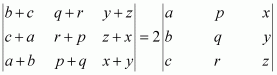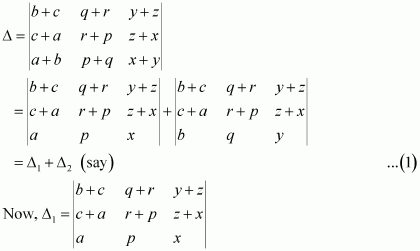Applying R2 → R2 − R3, we have: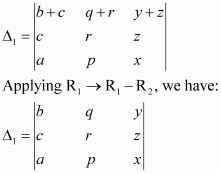Applying R1 ↔R3 and R2 ↔R3, we have: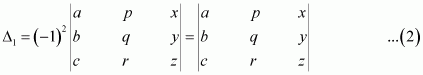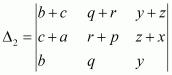Applying R→ R1 − R3, we have: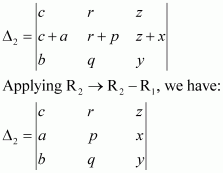Applying R1 ↔R2 and R2 ↔R3, we have: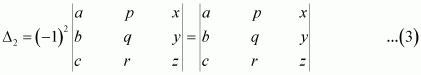From (1), (2), and (3), we have: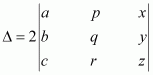Hence, the given result is proved.

#### Question 6:

By using properties of determinants, show that: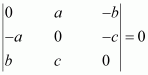We have,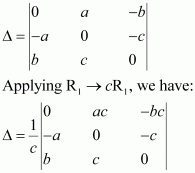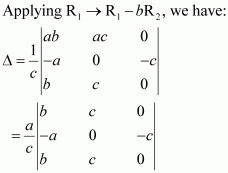Here, the two rows R1 and Rare identical.

Δ = 0.

#### Question 7:

By using properties of determinants, show that: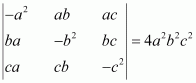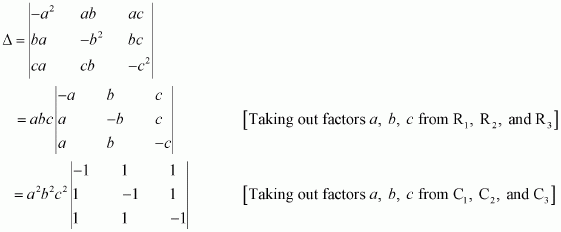Applying R→ R2 + R1 and R→ R3 + R1, we have: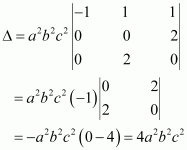#### Question 8:

By using properties of determinants, show that:

(i)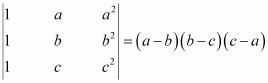(ii)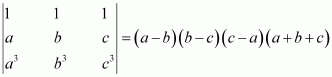(i)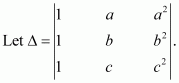Applying R1 → R1 − Rand R2 → R2 − R3, we have: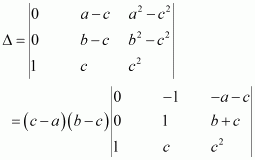Applying R1 → R1 + R2, we have: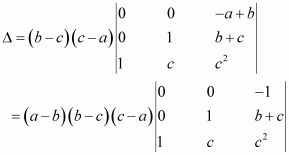Expanding along C1, we have: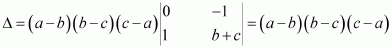Hence, the given result is proved.

(ii) Let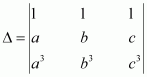.

Applying C1 → C1 − Cand C2 → C2 − C3, we have: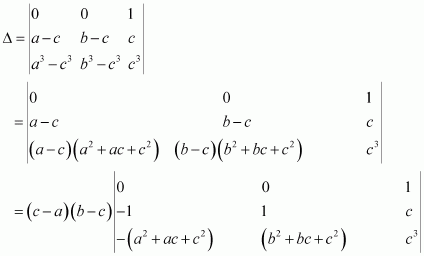Applying C1 → C1 + C2, we have: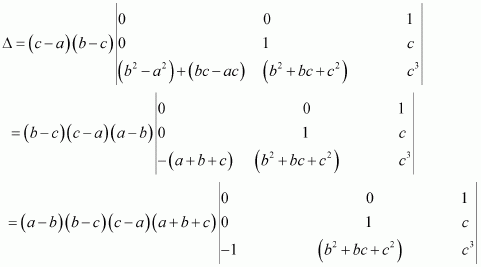Expanding along C1, we have: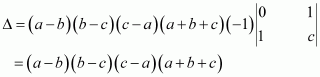Hence, the given result is proved.

#### Question 9:

By using properties of determinants, show that: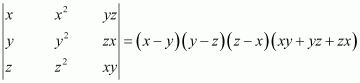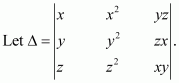Applying R2 → R2 − Rand R3 → R3 − R1, we have: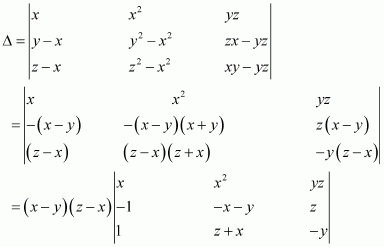Applying R3 → R3 + R2, we have: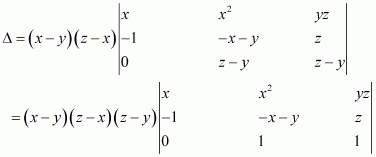Expanding along R3, we have: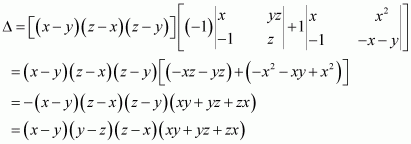Hence, the given result is proved.

#### Question 10:

By using properties of determinants, show that:

(i)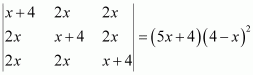(ii)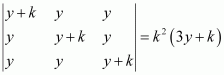(i)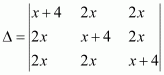Applying R1 → R1 + R+ R3, we have: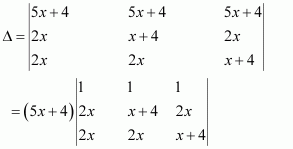Applying C2 → C2 − C1, C3 → C3 − C1, we have: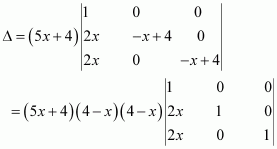Expanding along C3, we have: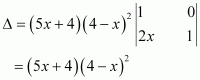Hence, the given result is proved.

(ii)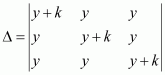Applying R1 → R1 + R+ R3, we have: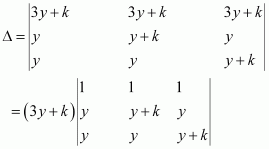Applying C2 → C2 − Cand C3 → C3 − C1, we have: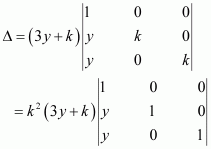Expanding along C3, we have: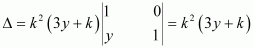Hence, the given result is proved.

#### Question 11:

By using properties of determinants, show that:

(i)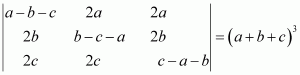(ii)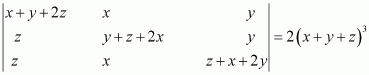(i)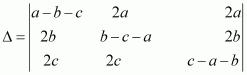Applying R1 → R1 + R+ R3, we have: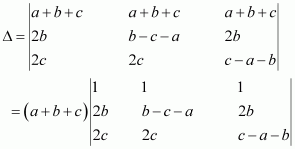Applying C2 → C2 − C1, C3 → C3 − C1, we have: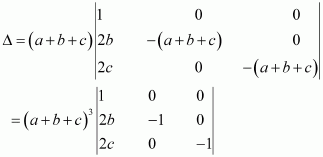Expanding along C3, we have: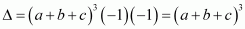Hence, the given result is proved.

(ii)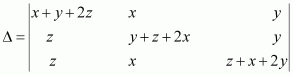Applying C1 → C1 + C+ C3, we have: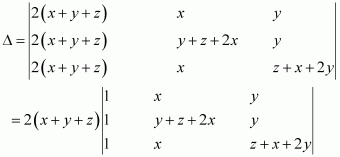Applying R2 → R2 − Rand R3 → R3 − R1, we have: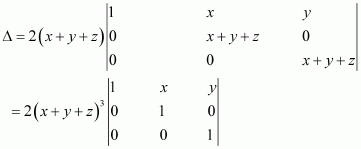Expanding along R3, we have: